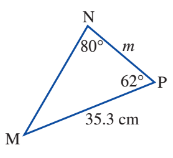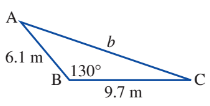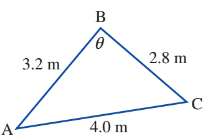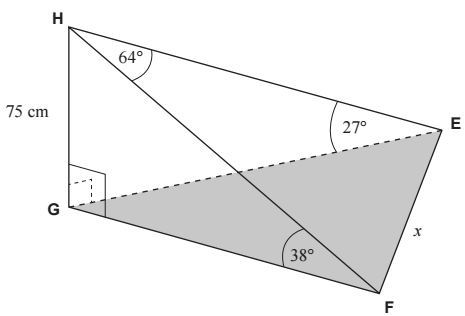Sine and Cosine Rule Diagnostic
Find the value of m correct to two decimal places.
1 pointFind Beta in the following triangle to the nearest degree.
1 pointFind the length of b in the following triangle correct to 1 decimal place
1 pointFind the value of theta to the nearest degree.
1 pointGiven the three-dimensional diagram below, determine the value of x.
1 point